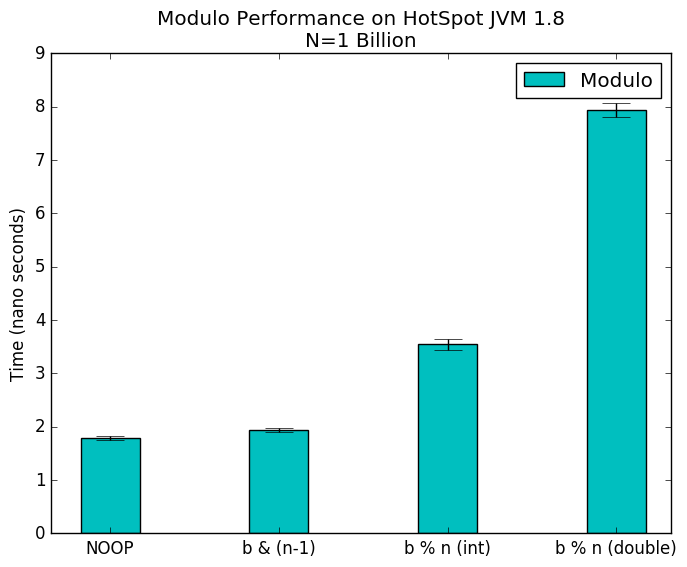I was surprised when someone told me not to use the modulo operator in high performance code. My textbooks used modulo (`%`) and various high performance implementations say to use modulo. Where had I gone wrong?

If you look at the JDK’s `mod()` implementation, you’ll see that it’s indeed `O(n)` for IEE754 floats, and `O(1)` for doubles. Note, the Java Spec defines modulo for integers and also for negative floating point numbers.

Luckily, there are tricks for modulo with integers.

## High Performance Modulo

Here’s the trick; don’t use floats. Floats are a pain for numerous reason, but let’s assume you’re wise enough to use a primitive integer type (int or long) to feed you `mod()` code, such as your hashmap implementation. There are many neat bit twiddling tricks to quickly conjure `mod(int,int)`.

### Powers of Two

Computers are binary by nature, so using powers of two provide lots of tricks. These can compute `mod(int, somePowerOf2)` in only a single machine instruction! Here’s how.

For example, if I want to do `61 % 8`, to know which of an `Array[Byte]` to grab a value from, we can think of it in binary as logical conjunction of the dividend with the mask of all bits lower than the divisor. For powers of 2, that’s just n-1. The bit operations are illustrated below, using 32 bit integers.

We can code this simply as:

``````def modPow2(n:Int, p2: Int) = n & (p2-1)

def isPow2(n:Int):Boolean = ((n-1) & n ) == 0

def modFast(n:Int, b: Int) = if (isPow2(b)) modPow2(n,b) else n % b

// The old way
def modOld(n:Int, b:Int) = n % b
``````

## Performance Comparsion

Comparing the byte code of classic modulo, and our faster version, we see they are both 4 lines of byte code. However, classic `%` calls the byte code operation `irem` which itself calls a native routine, so it’s far more complicated and won’t run in constant time.

``````  public int modOld(int, int);
Code:
2: irem
3: ireturn

public int modPow2(int, int); // with 8-1 inlined
Code:
1: bipush        7
3: iand
4: ireturn
``````

A simple benchmark1 on bare metal2 shows what we expect, doing one billion passes of each.

• Power 8 trick is the fastest
• `irem` implementation is nearly as fast
• `fmod` implementation is 3x slower

## Conclusions

• Modulo isn’t a major performance hog
• Avoid Double modulo operations if possible (can you use int’s?)
• The reference Java modulo implementation is fast
• For `mod(a,2^n)` operations, `modPow2` is ~23% faster than stock moduolo

If modulo is a critical path in your code, and your divisor is a power of 2 (with Natural dividends), use this trick. Otherwise, the stock JVM implementation should serve you well.

1. Benchmarked with a simple iterator, and ScalaMeter, and JMH (see repo). JMH provided the most consistent approach, and uses the most advanced methods to warmup and prevent garbage collections. Performed on an untilized, bare metal machine with N=1 billion (1K runs of 1M iterations). JVM memory preallocated at startup (`-Xmx=2G -Xms=2G`) [return]
2. HotSpot 1.8.0_91, Ubuntu 15.04, i7-4790K, 32GB PC3 19200 ram, SSD [return]